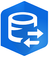# How can I compare one row of data to the row that came before and the row that came after it.

2000
18
09-07-2018 11:55 AMNew Contributor

I have a large group of gps points that was collected. I want to select all points that have a spar depth that is greater or less than 20% that of the points that came before or after it (based on the GISID).

GISID      Spar Depth

2022         4.035

2023         4.066

2024         3.604

2025         3.576

2026         4.593

2027         2.690

2028         2.960

2029         4.59

import arcpy
counter =0
with arcpy.da.SearchCursor('GPS Gas Main Pipe Point',[ 'GISID', 'SPAR_DEPTH']) as cursor:
for row in cursor:
MSDepth = row
if counter > 0:
diff = abs(MSDepth-oldMSDepth)
if diff > 20:
print('GISID {}'.format(row))
oldMSDepth = row
oldGISID = row
counter+= 1

Tags (4)
18 RepliesbyMVP Regular Contributor

Sounds like a job for numpy array:  FeatureClassToNumPyArray.  Right, Dan Patterson‌?byMVP Honored Contributor

See example #2 here for how to read all records into a list, then access items by index (or similarly read into a dictionary and access by key): SearchCursor—Data Access module | ArcGIS DesktopbyMVP Honored Contributor

If I understand correctly, you can load your data into a list and select those that return 'true':

``````l = [4.035, 4.066, 3.604, 3.576,  4.593, 2.690, 2.960, 4.59]

def compare(val_1, val_2, cur):
diff = abs(val_2 - val_1)
thresh = cur * 0.2
diff_minus_thresh = diff - thresh
if diff_minus_thresh > 0:
sel = True
else:
sel = False
return (l[i], diff, thresh, diff_minus_thresh, sel)

print('COMPARE BACKWARD')
for i in range(1, len(l)):
print(compare(l[i-1], l[i], l[i]))

print('COMPARE FORWARD')
for i in range(0, len(l)-1):
print(compare(l[i], l[i+1], l[i]))

COMPARE BACKWARD
(4.066, 0.030999999999999694, 0.8132, -0.7822000000000003, False)
(3.604, 0.46199999999999974, 0.7208000000000001, -0.25880000000000036, False)
(3.576, 0.028000000000000025, 0.7152000000000001, -0.6872, False)
(4.593, 1.017, 0.9186000000000001, 0.09839999999999982, True)
(2.69, 1.903, 0.538, 1.365, True)
(2.96, 0.27, 0.592, -0.32199999999999995, False)
(4.59, 1.63, 0.918, 0.7119999999999999, True)
COMPARE FORWARD
(4.035, 0.030999999999999694, 0.807, -0.7760000000000004, False)
(4.066, 0.46199999999999974, 0.8132, -0.3512000000000003, False)
(3.604, 0.028000000000000025, 0.7208000000000001, -0.6928000000000001, False)
(3.576, 1.017, 0.7152000000000001, 0.30179999999999985, True)
(4.593, 1.903, 0.9186000000000001, 0.9843999999999999, True)
(2.69, 0.27, 0.538, -0.268, False)
(2.96, 1.63, 0.592, 1.0379999999999998, True)

‍‍‍‍‍‍‍‍‍‍‍‍‍‍‍‍‍‍‍‍‍‍‍‍‍‍‍‍‍‍‍‍‍‍‍‍‍‍‍‍‍‍‍‍‍‍‍‍‍‍‍‍‍‍‍‍‍‍‍‍‍‍‍‍‍‍‍‍‍‍‍‍‍‍‍``````byMVP Legendary Contributor

Not sure exactly what you mean, but compare this to the answer you got for your example.  a0 should be the forward comparison and a1 the backward comparison.  If you see the answers are correct, I can finish it off with selecting from the input array, a, the index that meets one or both conditions.  That is the part that needs clarification.

I presume then, that you just and the GISID of the entries(?)

``````a = np.array([4.035, 4.066, 3.604, 3.576,  4.593, 2.690, 2.960, 4.59])

a0 = np.diff(a)/a[1:]*100

a1 = np.diff(a)/a[:-1]*100

a
array([4.035, 4.066, 3.604, 3.576, 4.593, 2.69 , 2.96 , 4.59 ])

a0
array([  0.76242007, -12.8190899 ,  -0.78299776,  22.14239059,
-70.74349442,   9.12162162,  35.51198257])

a1
array([  0.76827757, -11.36251845,  -0.77691454,  28.43959732,
-41.43261485,  10.03717472,  55.06756757])‍‍‍‍‍‍‍‍‍‍‍‍‍‍‍‍``````New Contributor

I am not sure something like this would work, I am running this script on 200,000+ gps points. I want to create some type of loop that looks at each point and compares the spar depth to the spar depth of the point collected before it and the point collected after it using the GISID to determine relationship.

My end goal is to select all points that has a spar depth that has a +-20% difference compared to the points collected before/after it.byMVP Legendary Contributor

My example is exactly what it does, compares the one before to the one after and check the difference.

If it agrees with your example, I can finish up the extra couple of lines that will append the resultant to your input table... unless of course you have the Data Interoperability extension and want to try that route firstbyEsri Regular Contributor

Hi, Data Interoperability extension has native adjacent feature inspection handling, this is in the AttributeManager transformer, up to 100 prior or subsequent features.byMVP Legendary Contributor

``````gisid = np.array([2022, 2023, 2024, 2025, 2026, 2027, 2028, 2029])
a = np.array([4.035, 4.066, 3.604, 3.576,  4.593, 2.690, 2.960, 4.59])
#
# ---- get the differences and put them into an array (z)
#
a0 = np.diff(a)/a[1:]*100
a1 = np.diff(a)/a[:-1]*100
z = np.zeros((8,4), 'float')
z[:, 0] = a
z[1:, 1] = a0
z[:-1, 2] = a1
z[:, 3] = ((abs(z[:,1])>20.) | (abs(z[:,2])> 20.)).astype('float')
#
# ---- make an output array that can be joined to the input table
#
names = ['GISID', 'Spar_depth', 'A0', 'A1', 'Bool']
vals =[gisid, z[:,0], z[:,1], z[:,2], z[:,3]]
dt_kind = ['i4', '<f8', '<f8','<f8','<i4']]
dt = np.dtype(list(zip(names, dt_kind)))
out = np.recarray((8,), dtype=dt)
for n in range(len(names)):
nme = names[n]
out[nme] = vals[n]
#
# ---- here is the output ready for joining to the input table
#
out
rec.array([(2022, 4.035,   0.        ,   0.76827757, 0),
(2023, 4.066,   0.76242007, -11.36251845, 0),
(2024, 3.604, -12.8190899 ,  -0.77691454, 0),
(2025, 3.576,  -0.78299776,  28.43959732, 1),
(2026, 4.593,  22.14239059, -41.43261485, 1),
(2027, 2.69 , -70.74349442,  10.03717472, 1),
(2028, 2.96 ,   9.12162162,  55.06756757, 1),
(2029, 4.59 ,  35.51198257,   0.        , 1)],
dtype=[('GISID', '<i4'), ('Spar_depth', '<f8'), ('A0', '<f8'),
('A1', '<f8'), ('Bool', '<i4')])#

#---- in your table in *map or *Pro, simply do a query on the Bool column‍‍‍‍‍‍‍‍‍‍‍‍‍‍‍‍‍‍‍‍‍‍‍‍‍‍‍‍‍‍‍‍‍‍‍‍‍‍‍‍‍‍‍‍‍‍‍‍‍‍‍‍‍‍‍‍‍‍‍‍‍‍‍‍‍‍‍‍‍‍‍‍‍‍‍‍‍‍``````byMVP Esteemed Contributor

I am not clear what percentage you are interested in applying the 20% threshold to in your question.  Using GISID 2024 as an example, are you interested in 2024 being 11.4% lower than 2023 or 2023 being 12.8% higher than 2024?  If your threshold was 12% instead of 20%, would 2024 be selected based on 2023 or not?